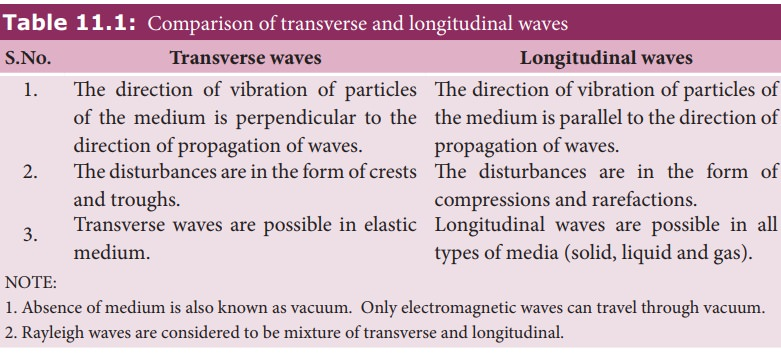Home | | Physics 11th std | Mechanical wave motion and its types

# Mechanical wave motion and its types

Wave motion can be classified into two types

Mechanical wave motion and its types

Wave motion can be classified into two types

a. Mechanical wave – Waves which require a medium for propagation are known as mechanical waves.

Examples: sound waves, ripples formed on the surface of water, etc.

b. Non mechanical wave – Waves which do not require any medium for propagation are known as non-mechanical waves.

Example: light

Further, waves can be classified into two types

a. Transverse waves

b. Longitudinal waves

Transverse wave motionIn transverse wave motion, the constituents of the medium oscillate or vibrate about their mean positions in a direction perpendicular to the direction of propagation (direction of energy transfer) of waves as shown in Figure 11.6.

Example: light (electromagnetic waves)

Longitudinal wave motion

In longitudinal wave motion, the constituent of the medium oscillate or vibrate about their mean positions in a direction parallel to the direction of propagation (direction of energy transfer) of waves as shown in Figure 11.7.

Example: Sound waves travelling in air.Study Material, Lecturing Notes, Assignment, Reference, Wiki description explanation, brief detail
11th Physics : UNIT 11 : Waves : Mechanical wave motion and its types |